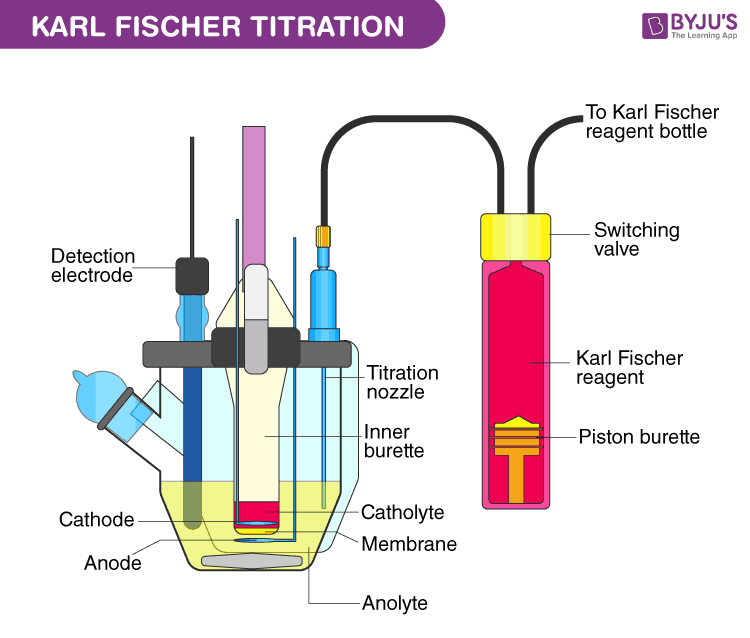Jet Set Go! All about Aeroplanes Jet Set Go! All about Aeroplanes

# Karl Fischer Titration Questions

Karl Fischer titration is a method used to determine the water concentration in a given analyte sample by using coulometric or volumetric titration. German chemist Karl Fischer uncovered it in 1935. It is based on the oxidation reaction between iodine and sulphur dioxide. Iodine and sulphur dioxide are hydrolysed to form hydrogen iodide and sulphur trioxide. The reaction ends when all the water molecules are consumed.

I2 + SO2 + H2O → 2 HI + SO3

 Definition: Karl Fischer titration is a method used to determine the water concentration in a given analyte sample by using coulometric or volumetric titration.

## Karl Fischer Titration Chemistry Questions with Solutions

Q1. What is the primary principle of the Karl Fischer Titration?

(a) To determine the boiling point of a given analyte

(b) To determine the moisture content of a given analyte

(c ) To determine the ash value of a given analyte

(d) To determine the optical rotation of a given analyte

Answer: (b) The primary principle of the Karl Fischer Titration is to determine the moisture content of a given analyte.

Q2. Which of the following can be used as a reagent in the Karl Fischer titration?

(a) Iodine, sulfur dioxide and a solvent

(b) Chlorine, nitrogen dioxide and a solvent

(c ) Both (a) and (b)

(d) None of the above

Answer: (a) Iodine, sulfur dioxide and a solvent can be used as a reagent in Karl Fischer titration.

Q3. Which of the following can be used as a buffering agent in the Karl Fischer titration?

(a) Pyridine

(b) Methanol

(c ) Both (a) and (b)

(d) None of the above

Answer: (a) Pyridine can be used as a buffering agent in the Karl Fischer titration.

Q4. Which of the following can be used as a solvent in the Karl Fischer titration?

(a) Pyridine

(b) Methanol

(c ) Both (a) and (b)

(d) None of the above

Answer: (b) Methanol can be used as a solvent in the Karl Fischer titration.

Q5. Which of the following is the correct chemical equation for the reaction between iodine and sulphur dioxide?

(a) I2 + NO2 + H2O → 2 HI + NO3

(b) Cl2 + SO2 + H2O → 2 HCl + SO3

(c ) I2 + SO2 + H2O → 2 HI + SO3

(d) Cl2 + NO2 + H2O → 2 HCl + NO3

Answer: (c ) I2 + SO2 + H2O → 2 HI + SO3 is the correct chemical equation for the reaction between iodine and sulphur dioxide.

Q6. What is Karl Fischer’s titration?

Answer: Karl Fischer titration is a method used to determine the water concentration in a given analyte sample by using coulometric or volumetric titration. German chemist Karl Fischer uncovered it in 1935.

Q7. What is the primary principle of the Karl Fischer Titration?

Answer: The primary principle of the Karl Fischer Titration is to determine the moisture content of a given analyte. It is based on the oxidation reaction between iodine and sulphur dioxide. Iodine and sulphur dioxide are hydrolysed to form hydrogen iodide and sulphur trioxide. The reaction ends when all the water molecules are consumed.

I2 + SO2 + H2O → 2 HI + SO3

Q8. Why is methanol used as a solvent in the Karl Fischer Titration?

Answer: Methanol is used as a solvent in the Karl Fischer Titration because it is polar in nature and is the most volatile alcohol.

Q9. What is DST? What is it used?

Answer: DST is the abbreviation for disodium tartrate dihydrate. It is primarily used for the standardisation of Karl Fischer’s Titration. Under standard conditions, DST is stable and non-hygroscopic.

Q10. Mention some factors that affect the Karl Fischer Titration?

Answer: Karl Fischer titration is a method used to determine the water concentration in a given analyte sample by using coulometric or volumetric titration. Various factors that affect the Karl Fischer Titration are mentioned below.

1. Humidity

2. pH of sample

3. Medium

4. KF reagent

Q11. What are the pieces of equipment of the Karl Fischer Titration?

Answer: Various pieces of equipment of the Karl Fischer Titration are mentioned below.

1. Drying tube

2. KF reagent

3. Sample injection cap

4. Anode chamber

5. Electrode analysis

6. Rotor

7. Cathode chamber

8. Drain cook

9. Detection electrode

10. Magnetic stirrerQ12. What is the primary discrepancy between coulometric and volumetric titration?

Answer: The primary difference between coulometric and volumetric titration is that in the coulometric titration, the titrant is produced electrochemically inside the titration cell. In contrast, in the volumetric titration, the titrant is added directly to the sample with the help of a burette.

Q13. What is the limit of the Karl Fischer factor?

Answer: The value of the Karl Fischer factor should not be less than 5 mg and more than 7mg.

Q14. What is the primary difference between Karl Fischer’s titration and loss on drying?

Answer: Both Karl Fischer’s titration and loss on drying are used to determine the water concentration in a given analyte sample by using coulometric or volumetric titration. But, loss on drying can also determine the volatile matter in a given analyte sample.

Q15. Match the following.

Column A

Column B

Solvent

Platinum

Buffering Agent

Iodine, sulfur dioxide and a solvent

Electrode

Pyridine

Reagent

Equipment

Detection electrode

Methanol

Column A

Column B

Solvent

Methanol

Buffering Agent

Pyridine

Electrode

Platinum

Reagent

Iodine, sulfur dioxide and a solvent

Detection electrode

Equipment

## Practise Questions on Karl Fischer Titration

Q1. What are the applications of Karl Fischer’s Titration?

Q2. What are the advantages of Karl Fischer’s Titration?

Q3. What are the limitations of Karl Fischer’s Titration?

Q4. What can you determine the water equivalence factor?

Q5. What is Karl Fischer’s Titration in pharma?

Click the PDF to check the answers for Practice Questions.